## research papersBIOLOGICALCRYSTALLOGRAPHY
ISSN: 1399-0047
Volume 57| Part 10| October 2001| Pages 1435-1444

## Fast Fourier feature recognition

aDepartment of Chemistry, University of York, Heslington, York YO10 5DD, England
*Correspondence e-mail: cowtan@yorvic.york.ac.uk

(Received 19 April 2001; accepted 26 June 2001)

Various approaches have been demonstrated for the automatic interpretation of crystallographic data in terms of atomic models. The use of a masked Fourier-based search function has some benefits for this task. The application and optimization of this procedure is discussed in detail. The search function also acquires a statistical significance when used with an appropriate electron-density target and weighting, giving rise to improved results at low resolutions. Methods are discussed for building a library of protein fragments suitable for use with this procedure. These methods are demonstrated with the construction of a statistical target for the identification of short helical fragments in the electron density.

### 1. Fast Fourier feature recognition

#### 1.1. Background

The identification of protein features is a key step in the interpretation of the data from a diffraction experiment. This feature-detection process takes many different forms dependent on the nature of the data available. If phases and, therefore, an electron-density map are unavailable, then the primary means for structure solution is molecular replacement. The entire molecule, or a large substructure, must be recognized from an appropriate model by matching against the observed structure-factor magnitudes.

Commonly, no such isomorphous model is available, in which case some sort of phase information is required. This phase information may be used to calculate a best' electron-density map. Feature recognition commonly relies on these maps, although a better approach would make simultaneous use of both the phase information and the unphased magnitudes.

Feature recognition in electron-density maps also takes various forms depending on resolution and map quality. At high resolution it is sufficient to perform a simple peak-picking procedure to identify atomic sites, as demonstrated in direct-methods programs such as MULTAN (Germain et al., 1970) or in a more sophisticated form in the ARP automated refinement procedure of Lamzin & Wilson (1997).

At lower resolutions it is common to trace ridges in the density to produce a skeleton', for example using the method of Greer (1985). This skeleton was initially used as an aid to visualization, with the user identifying protein motifs from the pattern of ridges; however, more recently the skeleton has been used as a basis for automated feature recognition, for example Jones & Kjeldgaard (1997) and the QUANTA (Molecular Simulations Inc., 2000) software. The approach is fast and effective; however, it does suffer from a significant limitation The topology of the electron density changes very quickly as the resolution of the map changes. Therefore, the methods used for interpreting the skeleton must change as the resolution of the density map changes. The ARP/wARP build' procedure of Perrakis et al. (1999), which fits pseudo-atoms into a map and traces protein-like motifs through them, has similar strengths and limitations.

An alternative approach which avoids this difficulty is to use the electron density of some expected protein fragment as a search model. The resolution of the search model may therefore be matched to the resolution of the map, yielding a method which will work at any resolution, given a suitably sized search fragment. At higher resolutions it may be possible to use small clusters of atoms, such as individual residues or pairs of residues, as search fragments. In this work, the problem of identifying common secondary-structure features, and in particular α-helices, in lower resolution maps is considered.

The identification of density motifs in an electron-density map requires that the search density be compared against the electron-density map at every possible position in the unit cell, for every possible orientation of the search density. This is a six-dimensional search over three translation and three orientation parameters, which is only practical computationally with careful optimization.

Kleywegt & Jones (1997) implemented an exhaustive real-space search with a molecular fragment by adopting a computationally simple target function. In the Essens' program a search was performed in six dimensions over all possible positions and orientations of a fragment. The fragment coordinates were mapped into the electron-density map using each possible orientation and translation in turn and the map densities near atomic centres were compared in order to obtain a score for that combination of orientation and translation. The best matches were stored and could be interpreted as a map of likely positions of the fragment in the map.

An alternative approach to the computational problem is to perform a three-dimensional search over orientations and then for each orientation consider every translation simultaneously by use of a Fourier-based translation function. The simplest function to implement by Fourier methods is the overlap integral, often called a phased translation function, given by the product of the fragment density and the map density summed over the volume of the fragment at each translation. (The summation is actually calculated over the whole map, since the fragment density will be zero beyond the boundaries of the fragment.) This function is easily calculated in reciprocal space by means of the convolution theorem.

Colman et al. (1976) suggested the use of this function for the location of an oriented molecular-replacement model using low-resolution phase information. The phased translation function was extended by Read & Schierbeek (1988) to form a simple correlation function. In the present work, a weighted mask function is also used to further improve the search function and allow its development into a simple statistical form.

#### 1.2. Fast Fourier feature recognition

Cowtan (1998b) described how a modified phased translation function could be efficiently calculated using FFTs and used to locate small atomic models in an electron-density map. By searching over all possible orientations of the fragment, all instances of the search fragment in the electron-density map could be identified.

Let the translation search function, which gives the agreement between the search fragment (in the current orientation) and the electron density as a function of fragment position, be called t(x). The fragment density is ρf(x) and the corresponding fragment mask is μf(x). The search function is then formed from the sum of the mean-squared difference in density between the offset fragment and the map,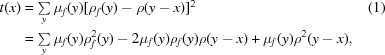where ρ is the best' electron-density map (typically a weighted Fourier map based on the observed magnitudes, phase estimates and figures-of-merit) and y is a coordinate used to sum over the volume of the fragment. An RMS difference may be formed from the square root of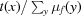if required.

Note that in the expansion the first term is independent of x and so is only calculated once, whereas the second two terms are convolutions and may therefore be efficiently calculated in reciprocal space as follows,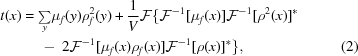where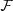represents the Fourier transform,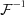the inverse Fourier transform and * complex conjugation. If the Fourier coefficients of the density and squared density are pre-calculated, then the translation function for a fragment in multiple orientations may be calculated by three fast Fourier transforms (FFTs) per orientation. Since the fragment will usually have no symmetry, all FFTs must be performed in P1.

Once the translation function has been calculated for a particular orientation of the search model, the results must be stored for combination with results from other orientations. This may be achieved by a peak search or by storing a map of best orientations' for each position in the unit cell as described by Kleywegt & Jones (1997) (this assumes that a fragment can only adopt one matching orientation at any location in the cell and that the fragment is positioned at the origin).

This method was implemented by Cowtan (1998b) and shown to provide similar accuracy with twofold to fivefold speed improvement over the real-space approach of Kleywegt & Jones (1997) for search models of 25–50 atoms.

#### 1.3. Optimization

The method outlined above can be improved upon in several ways to provide dramatic improvements in computational efficiency. These optimizations are as follows.

 (i) Rotation of the search model in reciprocal space. Instead of calculating the Fourier transform of the fragment mask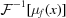and of the product of the mask and model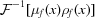for each search orientation, the Fourier transforms may be calculated once and the rotations performed in reciprocal space, in an equivalent manner to that used in a conventional rotation function; see for example Navaza (1987). The calculation required for each search orientation is therefore reduced from three FFTs to one. If the structure factors for the mask and mask × model are calculated on a fine orthogonal grid, then the rotation in reciprocal space can be calculated by linear interpolation for minimal computation overhead, giving a speed increase of close to threefold over the previous results. (ii) Use of crystallographic symmetry. While the translation search function itself does not obey crystallographic symmetry, the combination with the translation and orientation information must obey the crystal symmetry, since the arrangement of fragments in the true structure also obeys it. Crystallographic symmetry can therefore be utilized by calculating only a sub-region of the translation search function and generating the remaining regions from the results of other search orientations.In practice, it is simpler to use the crystallographic symmetry to reduce the number of search orientations. This is again performed in a manner analogous to a conventional rotation function, with the exception that the centrosymmetric property of a Patterson rotation function does not apply.If the search orientations are expressed in Eulerian angles, then the range of search orientations can be determined as in Table 1.NB is the order of rotational symmetry about the B axis; N⊥ is 2 if there are twofold axes perpendicular to the B axis and 1 otherwise. This makes optimal use of all primitive symmetries below cubic. Cubic and non-primitive symmetries will lead to some duplication of calculation.The use of space-group symmetry in this manner provides a fourfold speed increase over the P212121 calculations reported in Cowtan (1998b).In addition to use of the space-group symmetry, the metric for the Euler coordinate space should be used to ensure uniform sampling of orientation space, as described by Navaza (1987). (iii) Grid-doubling FFT. The peak-search step described in §1.2 depends on the translation function being calculated on a fine grid, otherwise peaks can be lost between grid points. In practice, the results are degraded if the grid spacing is greater than 0.2 times the map resolution (0.4 times the Nyquist spacing). Calculating the final FFT on such a fine grid would be time-consuming, so instead the FFT is performed on a grid at twice the desired spacing and the intermediate points obtained by interpolation. To avoid the translation-function peaks being eliminated by the smoothing effect of the interpolation, quadratic B-splines (Cowtan, 1998a) are used along with the corresponding spectral correction in reciprocal space. The interpolation may be performed along one dimension at a time; thus, only in the final dimension need all the points in the fine grid be considered. Convolution with the quadratic B-­spline interpolant at half-integral grid points can be performed with four floating-point operations; thus, the interpolation stage is not rate limiting and the calculation time is reduced by a factor close to 8,1 although in practice a slightly finer grid is used to ensure that no peaks are missed.
 Table 1Range of search orientations
Angle Range (°)
α 0–360/NB
β 0–180/N
γ 0–360

As a result of these optimizations, a fragment search on a 100-residue protein at 4.0 Å, as implemented in the FFFEAR program, typically takes 10–30 min on a modern workstation. This time is independent of fragment size and scales with FFT time for a given unit cell and resolution.

#### 1.4. Scaling issues

In order for the mean-squared difference residual to be effective in locating the search fragment in a map, the model and map density should be as consistent as possible. This means that the features in the fragment density should have the same scale and offset as those in the map and additionally that the features should be calculated at similar resolutions. The temperature factors of the fragment atoms should also be fitted to the map density, or alternately both map and fragment atoms can be sharpened to an arbitrary temperature factor, typically B = 0, using the method of Cowtan (1998a).

##### 1.4.1. Fragment resolution

Resolution may seem irrelevant for the fragment density, since the search function is actually assembled in reciprocal space and any higher resolution terms not available for the map may be discarded. However, the search function depends not on the Fourier transform of the fragment, but on the Fourier transform of the product of the fragment and the mask. As a result, it is found that better results are obtained when searching in low-resolution maps if the initial fragment density ρf(x) is calculated using resolution truncated atoms, generated using a spherically symmetric one-dimensional Fourier transform of the resolution-truncated atomic scattering factors.

##### 1.4.2. Fragment mean and scale: the use of density filters

The scale and offset of the fragment density can be dealt with by keeping all data on an absolute scale, but this ignores some practical problems. It is common for some low-resolution terms to be missing or unphased in experimental maps, leading to long-range ripples in the electron density across the unit cell. For small search fragments, these long-range variations in local mean density will add noise to the translation function; for large search fragments (e.g. molecular-replacement models) they can prevent any match from being found. This problem will be even worse in the case of NCS searches, where the search model is a reoriented volume of the map density and will therefore contain the same ripples running in a different direction.

Scale also becomes a problem when regions of the molecule exhibit different thermal motion: a copy of a fragment may be missed because its thermal motion is higher and the density peaks correspondingly lower. Again, this is a problem when searching for an NCS copy with higher thermal motion using density from a copy with lower thermal motion.

Cowtan (1998b) suggested matching the mean, and optionally the variance, of the search model and the map by use of a more complex search function (which becomes a correlation coefficient if both mean and variance are matched). This approach requires two additional FFTs and is less amenable to optimization than the simple mean-squared difference function.

An alternative approach is to pre-filter the map, and if necessary also the search model, to ensure that the local mean density calculated over a spherical region about each density point is constant. This achieves a similar result to the more complex search function; however, the filtering stage is performed once at the beginning of the calculation instead of requiring extra computation for every search orientation. This is equivalent to the mean adjusted residual of Cowtan (1998b), with the exception that the local mean is calculated over a spherical volume instead of the mask volume. Since the spherical volume is invariant under rotation, the filtering is factorized out of the orientation search.

If the filter sphere is chosen to be smaller than the resolution of any missing low-resolution data, then this approach additionally solves the problem of missing low-resolution terms, since their effect is largely removed from the map and the model. In practice, filtering the map and model densities with a sphere of radius 4–6 Å has proven to be vital when the volume of the search model is large, i.e. NCS and molecular-replacement calculations. Larger radii are required for lower resolution calculations.

#### 1.5. Statistical search function

A better indication of the presence of a particular fragment at a particular position in the unit cell may be obtained by use of a density-based statistical search function. This function may be constructed by calculating the probability that a particular electron-density map could arise from a given structural model and then applying Bayes' theorem to determine the probability of the model given the electron density.

The probability of a region of electron density taking on a particular conformation may be determined by sampling the electron density and taking the probability of obtaining a particular electron density at each sample point. This assumes that the electron density at each sample point is independent. This assumption is identical to that used by Terwilliger (1999) in the construction of a statistical solvent-flattening procedure and may be made under similar conditions, in particular that the resulting log-likelihood be rescaled so that the density of sample points in real space is equivalent to the density of independent reflections in reciprocal space. This assumption has been discussed further by Cowtan (2000).

In calculating the probability of an electron-density value at a particular position in the map on the basis of a fragment located at a particular translation, it is necessary to take into account the two following major sources of error.

 (i) The error in the map owing to errors in the phases and missing reflections in the data set. (Missing high-resolution terms may be ignored, however, if the search density is constructed at the correct resolution.) This gives rise to a scaling term, analogous to the D' term in the σA method of Read (1986), and a noise term. (ii) The error in the model density owing to the inherent variability in the structural motif to be located. In general, the search model will be at best an approximate match to one or more regions of the target molecule. The model density might be expected to be more reliable for the more central residues of the fragment and for main-chain rather than side-chain atoms.

The search function is constructed using Bayes' theorem, which may be written as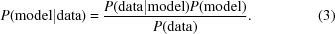In this case, the data is the electron-density map and the model is a specific placement of the current search fragment. Let F represent the case that the electron density arises from a correctly positioned and oriented fragment and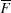represent the case that the electron density arises from any other source (i.e. incorrectly positioned fragment or density arising from a completely different model). The probability of a correctly positioned fragment given an individual density value from the map is then given by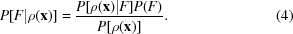P(F) is the prior probability of a particular fragment, position and orientation. When searching with a single fragment with no other prior information about the map, this will be a constant. Alternatively, information about solvent regions and interpreted density may be incorporated in the prior.

P[(ρ(x)] is the probability of the observed' map density at x. It may be calculated as a marginal distribution of P[ρ(x), C], C ∈ |F,|, i.e.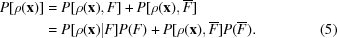For any complex fragment, it is far more likely that a density value will arise from any other source than from the correct fragment correctly oriented and positioned, therefore P() will dominate over P(F). Neglecting this first term, (4) becomes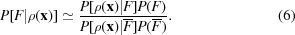The probability of an electron-density value given a particular correctly positioned fragment will be approximated by a Gaussian whose mean is the expected fragment density and whose variance is given by the variance of the distribution of densities at that position in the fragment over all matching fragments in the database. (The calculation of these density distributions is considered in §2.)

In order to account for noise in the electron-density map, this variance must be increased by the expected noise level in the map, given by Blow & Crick (1959),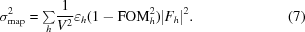The target density must also be scaled down by a factor D, analogous to D in the σA calculation,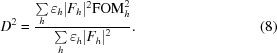The probability of an observed density value arising from a correctly positioned fragment is then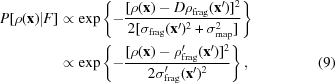where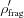(x′) = Dρfrag(x′),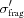(x′)2 = σfrag(x′)2 +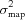and x′ is the coordinate in the fragment which maps to the point x in the map under the current translation and orientation of the fragment.

The probability of an observed density can be derived from the histogram of a typical protein density map at the given resolution, but it is simpler to make a Gaussian approximation using the fragment density map itself by simply choosing a position in the map where the atomic features are uncorrelated between matching fragments in the database (typically by taking a point distant from the centre of the fragment and outside of it). If the mean and variance of such uncorrelated density are given by ρrand and σrand, then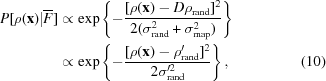where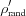= Dρrand and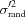=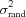+.

Substituting these expressions in (6) and discarding the constant terms gives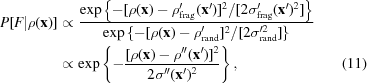where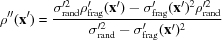and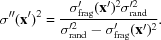Finally, the probability indications for the presence of a fragment on the basis of each individual density value in the map are combined to give an overall indication of the probability of the fragment being present with the current translation and orientation,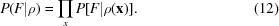It is more convenient to form the logarithm of this expression,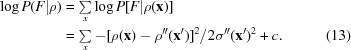This expression is of the same for as the FFFEAR mean-squared difference residual described in §1.2. Note, however, that the mask function becomes a continuously varying function in the form of an inverse variance and also that the target density has been modified according to the value of the mask function.

The resulting function may therefore be efficiently calculated using the FFT approach described earlier. However, to construct the target function it is necessary to have both mean and variance estimates of the search density as a function of position, with the variance providing an indication of the variability of the density at a particular position between fragments matching the desired overall configuration. The mask function based on this variance replaces the earlier binary mask, automatically masking a volume over which the fragment density is significantly better determined than random protein density.

The construction of appropriate statistical density targets is discussed in the following section.

### 2. Fragment clustering using the Protein Data Bank

In order to generate statistical search targets with accurate density statistics, or even conventional search-fragment models, it is necessary to analyse a large representative set of known structures. A set of common protein motifs for use as search models, along with frequency information, may be determined by analysis of the Protein Data Bank. The most common tool for the identification and classification of such motifs is cluster analysis, by means of which common features can be identified without the imposition of any prior prejudice concerning which motifs might be important. The basic cluster-analysis approach depends on pairwise comparison of all candidates and so is computationally convenient as long as the number of candidates to be compared is significantly less than 104.

In this work nine-residue fragments of polypeptide chain are considered, although the same analysis will work for longer and shorter fragments. The Protein Data Bank (Berman et al., 2000) currently contains around 15 000 structures, most of which contain hundreds of such fragments (counting every possible choice of nine contiguous residues from the structure); therefore, cluster analysis of all possible nine-residue fragments is impractical.

The first step in addressing this problem is to remove the redundant structures from the database. A representative set of well determined X-ray structures were selected from the database by the following method. The FSSP index (Holm & Sander, 1996) was used as a basis for selecting a subset of the database for analysis. This index divides the Protein Data Bank into representative chains and homologous sets of chains with greater than 30% sequence identity to each representative chain. From each homologous set, the X-ray structure determined at the highest resolution (if any) was selected as a representative of that set. Each representative X-­ray structure was divided into overlapping nine-residue fragments. The resulting data set consisted of 394 186 fragments from 2793 structures.

The number of fragments is still impractical for cluster analysis, so further steps must be taken to reduce the number of fragments to be compared.

#### 2.1. Pre-clustering the fragments

The number of comparisons may be further reduced by replacing groups of similar fragments by a single representative model. The number of individual fragments will increase as more structures are considered, whereas the the number of representative fragments will remain largely constant and depend only on the radius within which two fragments are considered equivalent. This combination of fragments again requires pairwise comparison of fragments; however, the problem can be addressed efficiently if the fragments are first broken down into groups with similar structural features. For this purpose only the Cα atoms are considered, thus each fragment is reduced to nine sets of coordinates in three dimensions.

This problem of breaking down the conformational space has been addressed by Oldfield (1992) by use of a hashing algorithm based on the pseudo-Ramachandran angles (i.e. the opening angles between three Cα atoms and the torsion between four Cα atoms). The pseudo-Ramachandran angles are calculated for the fragment and then quantized in steps of 20°, for example. The quantized angles can be represented by a list of small integers: 13 integers for a nine-residue fragment, or six if the opening angles are ignored. These integers are then combined in a systematic manner to produce a hash code – an integer value which depends on all of the quantized angles, although several combinations of angles may lead to the same hash code. The fragments are indexed by their hash codes and only those structures with the same hash code are compared with each other. Thus, all similar fragments should have the same hash code, but some dissimilar fragments may also match the same hash code. The dissimilar fragments are obvious once comparisons are made within the set.

One problem with this approach is that changes in the torsions along the fragment are cumulative and thus small changes in several angles which do not affect the quantized values can lead to significantly different structures. Oldfield (2000) suggested a related procedure using the elements of the distance matrix instead of the pseudo-Ramachandran angles. Since the distance matrix contains long-range as well as local conformational information, this avoids the cumulative effects of small changes. However, the distance matrix of nine Cα atoms contains 36 elements (or 15 if the 1–2 and 1–3 distances are neglected) and is thus highly redundant. Furthermore, the more parameters are involved in construction of a hash code, the more often a cluster of similar conformations will be split over a boundary in the quantization scheme.

#### 2.2. Eigensystem analysis of the Cα distance matrix

The Cα distance matrix uniquely identifies a Cα fragment, apart from an ambiguity of hand, by combining information about both short-range and long-range structural features. However, to be useful in the classification of fragment conformation, the distance matrix needs to be reparameterized in such a way as to remove the redundancy in the distance-matrix elements. This may be achieved by defining a new set of parameters which are linear combinations of the distance-matrix elements but which are not correlated in the same way as the distance-matrix elements.

The reparameterization is achieved as follows. The 36 unique elements of the distance matrix (assuming nine Cα atoms) are calculated for each nine-residue fragment in the database. These 36 values are converted into a 36-element vector for each fragment. The variance of each element and the variance of each pair of elements of the vector are calculated and used to form a 36 × 36 element variance–covariance matrix whose rows and columns correspond to the individual elements of the vector.

This matrix is then diagonalized using a standard eigenvalue calculation. The matrix of eigenvectors is a rotation matrix from the original coordinate system (the elements of the distance matrix) into the new coordinate system (the eigenparameters). The eigenvalues are then the variances of the eigenparameters.

The eigenvalue spectrum of the variance–covariance matrix of distance-matrix elements when calculated across a large number of fragments is shown in Table 2. Note that the spectrum is dominated by less than ten significant eigenvalues, with the remainder being small. This is a result of the high degree of redundancy in the distance matrix.

 Table 2The ten largest eigenvalues of the variance–covariance matrix of distance-matrix elements
Eigenvalue number Eigenvalue
1 149.53
2 25.45
3 11.63
4 7.49
5 3.92
6 2.81
7 2.30
8 1.45
9 1.29
10 0.7436 0.0024

It is interesting to examine the significance of the eigenparameters which vary most from fragment to fragment. To visualize the eigenparameters, representative fragments were chosen with extreme (high and low) values for one eigenparameter and values close to the mean for all the others. The representative fragments for the first four eigenparameters are shown in Fig. 1.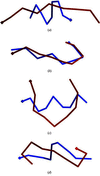Figure 1 Representative fragments for extreme values of the first four eigenparameters of the distance matrix. (a) is the eigenparameter corresponding to the largest eigenvalue, followed by (b), (c) and (d), respectively.

The first eigenparameter represents the extent' of the chain, with the extremes representing maximally extension or a compact ball of residues. The second eigenparameter determines whether the fragment is hooked' at either the start or the end of the chain. The third eigenparameter differentiates between linear' fragments, where the residues are arranged along a linear axis, and curved' fragments, where the residues arc away from the line connecting the ends. The fourth eigenparameter differentiates between fragments which are curved in the ends and those which are twisted in the middle.

Well known secondary-structure features can easily be identified from the first three eigenparameters: β-strands have large values of the first eigenparameter (indicating a large extent), whereas helices give rise to below average values of the first eigenparameter (indicating a short extent) and large values of the third (indicating no overall curvature).

In practice, for the classification of general fragments it was found to be more effective to use five eigenparameters corresponding to the largest eigenvalues. If these are quantized at equal intervals to divide the parameter space into roughly 104 regions, then each region is found to contain broadly similar conformations.

#### 2.3. Microclustering of fragments

Once the fragments have been classified by dividing them into regions of the eigenparameter space, then similar structures may be merged together to produce microclusters', with the aim that there will be few enough microclusters for analysis by a full clustering algorithm.

Each fragment in a region of eigenparameter space is considered in turn as a potential microcluster. The fragment is first compared with any previously formed microclusters in the region by rotating to a common orientation using the Kabsch algorithm (Kabsch, 1978) and then calculating an RMS coordinate difference. If the RMS difference in Cα coordinates between the current fragment and any previous microclusters in the region is less than some small value (0.5 Å was used for this work) then a weighted average is performed between the fragment and the microcluster, and the weight of the microcluster is incremented by 1. Otherwise, the fragment becomes a new microcluster of weight 1. This approach relies on the differences between the fragments within a microcluster being very small, otherwise the stereochemistry of the averaged structures will be unrealistic.

Whilst the number of fragments increases with the size of the database, the number of microclusters should remain constant once the conformation space is fully populated. Eliminating those microclusters with weights below some fraction of the size of the database (e.g. 10−4) removes unusual conformations without affecting the common motifs which are of interest.

#### 2.4. Cluster analysis of the microclusters

The remaining microclusters may be subjected to conventional cluster analysis. For each pair of microclusters, an RMS coordinate difference is again calculated to form a symmetric matrix whose indices are microcluster numbers. The two microclusters with the smallest separation are combined to form a new cluster whose weight is the sum of the weight of the two microclusters. The corresponding rows and columns of the matrix are combined by a weighted average using the microcluster weights. The resulting matrix has rank one less than the original matrix. This process is repeated, combining clusters and microclusters until a single cluster remains.

The order of the clustering is recorded, together with the weight of each cluster and the spacing between the two clusters from which it was formed. The important clusters may then be identified by choosing a desired cluster separation and examining the largest distinct clusters remaining at that point in the clustering process.

The principal motifs to emerge from this analysis, using a critical separation of 2.5 Å, include a helix motif, a curved strand motif, a combined helix + strand motif and a turn motif composed of two similar but distinct conformations. The actual cluster frequencies are strongly dependent on the exact cluster separation selected, suggesting that conformational space is fairly continuously populated in some directions, even if the population density is much higher in some regions. The frequency of the helix and strand motifs is also inflated by the fact that the same motif may repeat at one-residue intervals along the chain.

Smaller clusters contain straighter and more curved strands, some less common helix + coil and strand + coil motifs and the same turn motifs offset along the chain in either direction. However, there is no obvious separation of the helix motifs into clusters of different pitches at this cluster separation.

Any of these clusters may then be used to construct a statistical search model for use in the FFFEAR program. For each cluster, the mean and variance of the electron densities of all fragments in the cluster may be calculated for each point in a spherical region about the centre of the fragment. The mean and variance are then used to calculate the target density and weighting function (as described in §1.5). The mean electron density and the standard deviation of the electron density for the nine-residue helix cluster are contoured in Fig. 2. The mean density shows the features of the helix clearly, but only the stumps of the side chains. The density variance shows the conserved core of the fragment, with hollows around the Cα atoms arising from the variability of the side-chain density.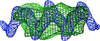Figure 2 Mean (blue) and standard deviation (green) of the electron densities across all helical fragments.

The sensitivity of a feature search using such a model will be affected by the critical cluster separation: if a large separation is chosen, more matches are likely to be found but the corresponding map features will be more diverse.

### 3. Results: fragment recognition

Comparisons between the basic FFFEAR search function and other methods are given in Cowtan (1998b). Further comparisons are provided here of the improvements to the basic search function described in this paper.

To test the modifications to the search function, the structure of O6-methylguanine-DNA methyltransferase (Moore et al., 1994), a DNA-repair protein of 178 amino acids, was used. The structure includes six helices and a three-strand β-sheet. The space group is P212121. All the data were available for three derivatives for this structure, allowing the calculation of maps of varying qualities by using different subsets of the data.

For the comparisons presented here, an MIR map was calculated using only two of the derivatives (mercury and lead), giving a mean figure of merit of 0.51 to 3.0 Å resolution. The resulting data was degraded by truncating the phases to 8.0 Å resolution: the map was therefore of higher quality than a genuine 8.0 Å MIR map, but still showed no detailed features and a high level of noise.

Feature searches were were performed over both translational and rotational spaces. The searches all used the nine-residue helix fragment obtained by analysis of the PDB; however, the density-search function was calculated in three different ways.

 (i) Using an electron-density model based on 8.0 Å resolution-truncated atoms and the fragment coordinates with no filtering. (ii) Using an electron-density model based on 8.0 Å resolution-truncated atoms and the fragment coordinates with a 8.0 Å radius filter to correct the mean density in the electron-density map. (iii) Using the statistical target density calculated directly from the ensemble of densities of matching fragments in the PDB at 8.0 Å without filtering.

The results are shown in Figs. 3(a), 3(b) and 3(c), respectively. The 50 best matches against the density are shown in each case. The horizontal axis gives the value of the FFFEAR fitting function for that calculation (low values represent a better estimated fit). The vertical axis is the RMS coordinate difference between the determined fragment orientation and the nearest segment of true chain (low values represent a better fit to the true structure). The symbols represent the conformation of the nearest matching segment of the true structure, with filled dots representing helices in the correct direction, open dots representing reversed helices and triangles for all other features.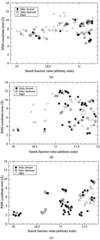Figure 3 Results of fragment search in 8 Å map using various search functions. The x axis gives the value of the search function for each match; the y axis gives the true RMS coordinate error to the nearest section of main chain. (a) Basic search function. (b) Basic search function with electron-density filtering. (c) Statistical search function with 8 Å target.

In Fig. 3(a) it can be seen that without filtering of the map density no correct matches are found. When density filtering is introduced (Fig. 3b) three correct matches are found to the best helix in the true structure; however, the remaining helices are lost amongst the noise peaks. The statistical target function in Fig. 3(c) further improves the results, with the proportion of correct matches being increased. Correct matches are found to three of the six helices in the structure before the first incorrect match, with a fourth appearing in the next cluster of results. Similar results are obtained with SIR maps at 6.0 Å resolution; however, at higher resolutions little benefit it seen over the conventional search function.

Once the search has been run, it is usually possible to filter out most of the incorrect solutions by selecting connected overlapping fragments and deleting inconsistently overlapping fragments. An automated utility, ffjoin', has been written for this purpose.

### 4. Conclusions

Methods for interpretation of crystallographic data in terms of protein structure have been considered. An electron-density-based search has the advantage that the search target can be calculated to match the changing topology of the density as a function of resolution. This technique has been implemented in the program FFFEAR and optimized to provide rapid results for small- to medium-sized proteins. The implementation of electron-density filtering improves the results of the method in the presence of noise and missing data.

The approach has been extended by the construction of a simple statistical search function which can be cast into the same form as the FFFEAR search function for efficient computation. Whilst this is not a full statistical treatment, since it ignores the information from unphased structure-factor magnitudes and is susceptible to phase bias, this approach has been shown to be beneficial at lower resolutions.

Methods have been described for the efficient identification of structural motifs in the Protein Data Bank. These have been demonstrated in the construction of a statistical target for the location of small helix fragments. It is hoped that the methods described may also prove useful in other data-mining problems.

### Footnotes

1Note that the same procedure may be applied in reverse for structure-factor calculation: density is calculated on a fine grid, convoluted with the spline function to obtain values at coarse grid sites, transformed and a spectral correction applied to the resulting structure factors. This is a particularly effective approach for the fast calculation of E values from coordinates, since the convolution of the spline function with a δ-function can be constructed analytically.

### ReferencesBIOLOGICALCRYSTALLOGRAPHY